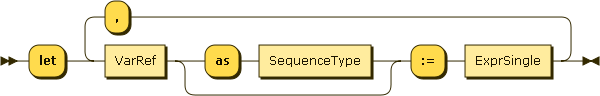### Let clauses

Figure 57. LetClauseLet bindings can be used to define aliases for any sequence, for convenience.

For each incoming tuple, the expression in the let clause is evaluated to a sequence. A binding is added from this sequence to the let variable in each tuple. A tuple is hence produced for each incoming tuple.

Example 131. An order by clause.

```for \$x in collection("captains")
let \$century := \$x.century
group by \$century
let \$number := count(\$x)
where \$number gt 1
return { "century" : \$century, "count" : \$number }
```

Result (run with Zorba): { "century" : 24, "count" : 4 }

Note that it is perfectly fine to reuse a variable name and hide a variable binding.

Example 132. An order by clause.

```for \$x in collection("captains")
let \$century := \$x.century
group by \$century
let \$number := count(\$x)
let \$number := count(distinct-values(for \$series in \$x.series
return typeswitch(\$series)
case array return \$series()
default return \$series ))
where \$number gt 1
return { "century" : \$century, "number of series" : \$number }
```

Result (run with Zorba): { "century" : 24, "number of series" : 3 }